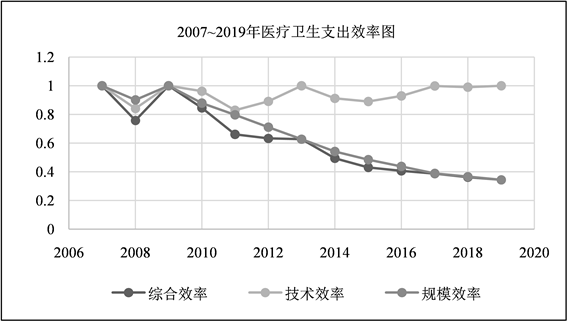#### 期刊菜单

Analysis on the Efficiency and Influencing Factors of Medical and Health Expenditure in Guizhou Province
DOI: 10.12677/AAM.2021.1011425, PDF, HTML, XML, 下载: 24  浏览: 52

Abstract: Based on the medical and health expenditure data of Guizhou Province from 2007 to 2019, this paper uses DEA data envelopment analysis method to measure the input-output efficiency of the government on medical institutions in each year, and establishes a grey relational model to analyze the factors that affect the efficiency of medical institutions in different regions in different periods. The results show that the medical and health efficiency of Guizhou province achieved a high DEA efficiency in 2007 and 2009, in which the overall efficiency and scale efficiency show a downward trend, while the technical efficiency remains at a high level. The factors that have great influence on medical efficiency are urbanization level, financial self-sufficiency rate, population density, farmers’ Engel coefficient, urban Engel coefficient and per capita GDP. Among them, the effect from urbanization level to per capita GDP gradually weakened.

1. 引言

2. 指标选取及数据来源

2.1. 数据来源

2.2. 指标选取

3. 基于三阶段DEA模型的财政医疗卫生支出效率分析

3.1. DEA模型

DEA (Data Envelopment Analysis)数据包络分析法最早由Charnes等学者提出，其基本原理是在输入或者输出不变的条件下，将各个决策单元投影到生产前沿面上，在前沿曲线上的决策单元具有最佳效率，效率值等于1；而不在前沿曲线上的决策单元属于DEA非效率，取值范围在0~1之间。本文基于DEA模型中的 ${C}^{2}R$ 模型，进行计算和分析。 ${C}^{2}R$ 模型设定决策单元数为n，每种决策单元都有m种输入和s种输出，将松弛变量 ${S}^{-}$、剩余变量 ${S}^{+}$ 和非阿基米德无穷小量 $\epsilon$ 加入线性规划可得：

$\left\{\begin{array}{c}\mathrm{min}\left[\theta -\epsilon \left({\sum }_{r=1}^{t}{s}_{r}^{+}+{\sum }_{i=1}^{m}{s}_{i}^{-}\right)\right]\\ s.t.{\sum }_{j=1}^{n}{X}_{j}{\lambda }_{j}+{s}^{-}+\theta {X}_{j0}=0\\ {\sum }_{j=1}^{n}{X}_{j}{\lambda }_{j}-{s}^{+}={Y}_{j0}\\ {\sum }_{j=1}^{n}{\lambda }_{j}=1\\ {\lambda }_{j}\ge 0,\text{\hspace{0.17em}}\text{\hspace{0.17em}}j=1,2,3,\cdots ,n\\ {s}^{+}\ge 0,\text{\hspace{0.17em}}\text{\hspace{0.17em}}{s}^{-}\ge 0\end{array}$ (1)

3.2. 灰色关联度模型

4. DEA数据描述及结果分析

4.1. DEA数据描述Table 2. The original data table of medical input and output in Guizhou Province

4.2. DEA效率结果分析Table 3. Medical and health expenditure efficiency results in Guizhou ProvinceFigure 1. Medical and health expenditure efficiency in Guizhou Province

5. 贵州省医疗支出效率影响因素的关联度分析

5.1. 数据描述Table 4. Raw data of factors affecting medical expenditures in Guizhou Province

5.2. 结果分析Table 5. Average processing results of correlation analysisTable 6. Analysis of relevance results

6. 政策建议

6.1. 合理划分财政收入

6.2. 推进全面绩效预算改革进程

6.3. 保证医疗卫生财政支出规模

6.4. 提高社会公众监督参与

  罗兴静, 王梦佳, 黎耀晖, 韦杰, 张科, 张丽, 汪俊华, 田娟. 2011-2017年贵州省卫生总费用筹资水平和筹资结构分析[J]. 医学与社会, 2020, 33(4): 70-74.  罗红雨. 我国省级财政卫生支出效率及影响因素实证研究[J]. 中国卫生经济, 2012, 31(6): 13-15.  程琳, 廖宇岑. 地方政府医疗卫生支出效率及其影响因素分析: 基于异质性随机前沿模型[J]. 中国卫生经济, 2015, 34(1): 16-18.  张凤, 任天波, 王俏荔. 公共医疗卫生支出效率及其影响因素研究——以宁夏为例[J]. 中国卫生事业管理, 2018, 35(6): 428-432.  孙玉凤, 任天波, 时保国, 王玉萍, 吕晓萌. 中国卫生支出效率的空间异质性及其影响因素研究[J]. 中国卫生事业管理, 2021, 38(2): 114-116+128.  周子超. 中国省级政府医疗卫生支出效率及其影响因素研究——基于新冠疫情背景下的反思[J]. 经济问题探索, 2021(2): 49-65.  李郁芳, 王宇. 中国地方政府医疗卫生支出效率及影响因素研究[J]. 海南大学学报(人文社会科学版), 2015, 33(3): 41-49.  季小雨, 姚育楠, 曾智. 基于灰色关联度分析方法的江苏省个人卫生支出影响因素研究[J]. 中国卫生经济, 2020, 39(11): 40-43.